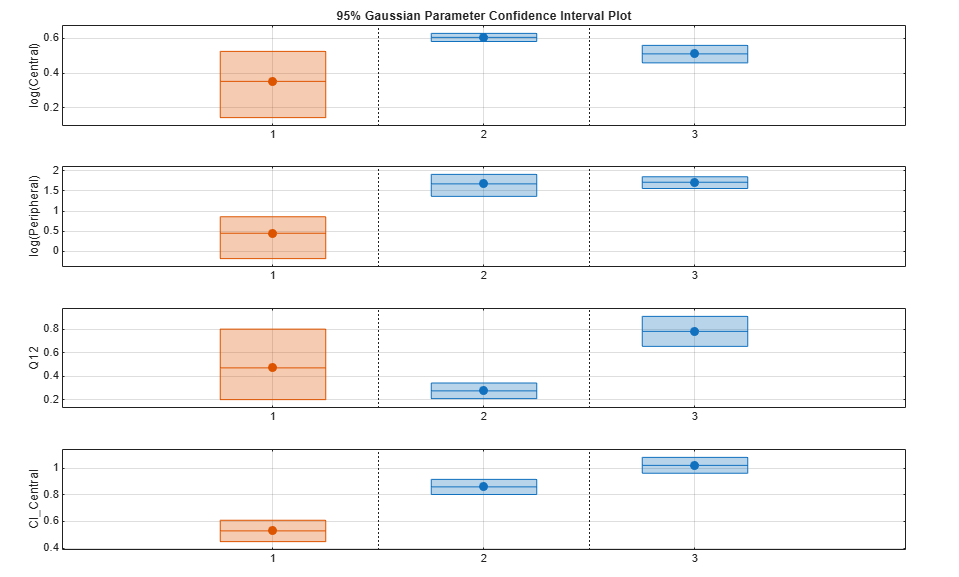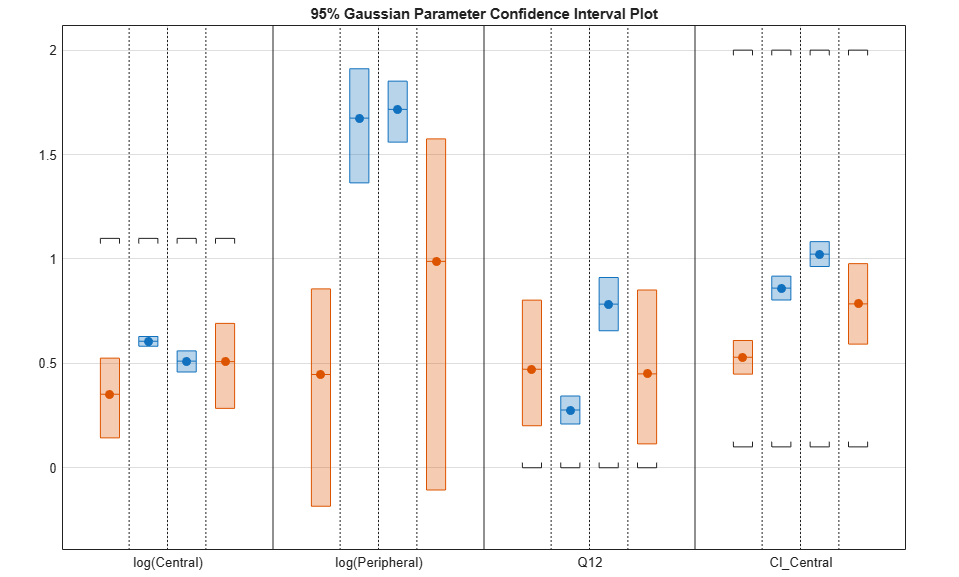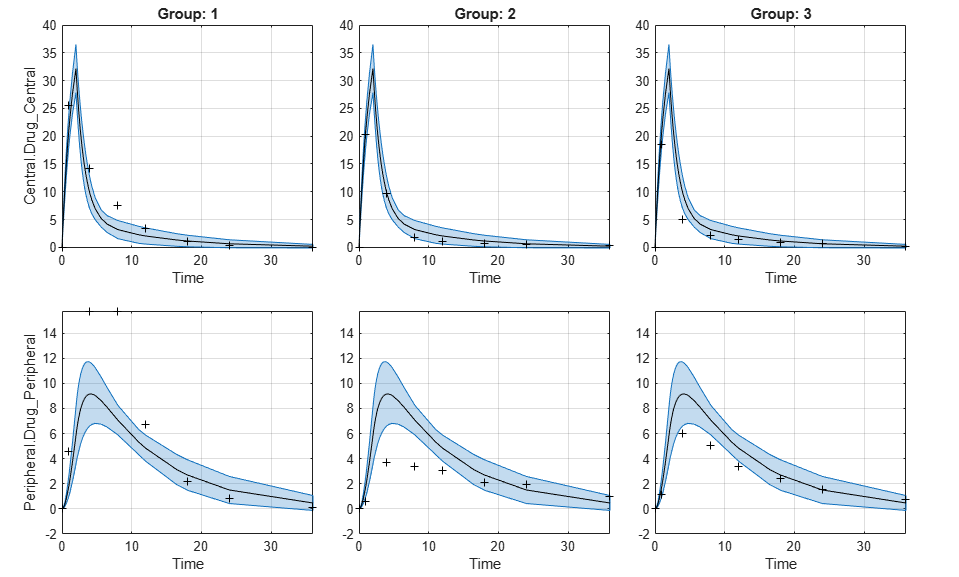Main Content

# PredictionConfidenceInterval

Object containing confidence interval results for model predictions

## Description

The `PredictionConfidenceInterval` object contains confidence interval results for model predictions (that is, simulation results based on estimated parameters).

## Creation

Create a prediction confidence interval object using `sbiopredictionci`.

## Properties

expand all

This property is read-only.

Names of model responses in the parameter fit, specified as a cell array of character vectors. Each cell contains the name of a response.

Example: ```{'Central.Drug_Central' } {'Peripheral.Drug_Peripheral'}```

This property is read-only.

Confidence interval estimation status, specified as one of the following categorical values:

• `success` – The proper confidence intervals are found. That is, no model prediction is constrained by the parameter bounds defined in the original fit.

• `constrained` – The confidence intervals are found, but the confidence interval for the model response is constrained by a parameter bound defined in the original fit.

• `not estimable` – No confidence intervals are found.

• `estimable` – The proper confidence intervals are found, but other model predictions have an estimation status of either `constrained` or ```not estimable```. For the bootstrap confidence interval, the status is always set to `estimable`.

Example: `success`

This property is read-only.

Confidence interval type, specified as `'gaussian'` or `'bootstrap'`.

Example: `'bootstrap'`

This property is read-only.

Confidence level, `(1-Alpha) * 100%`, specified as a positive scalar between 0 and 1.

Example: `0.01`

This property is read-only.

Original group names from the data used for fitting the model, specified as a cell array of character vectors. Each cell contains the name of a group.

Example: `{'1'}{'2'}{'3'}`

This property is read-only.

Confidence interval results, specified as a table. The table contains the following columns.

Column NameDescription
GroupGroup name
ResponseModel response name
TimeSimulation time
EstimateEstimated response value
ConfidenceIntervalConfidence interval values

This property is read-only.

Exit flags returned during the calculation of `bootstrap` confidence intervals only, specified as a vector of integers. Each integer is an exit flag returned by the estimation function (except `nlinfit`) used to fit parameters during bootstrapping. The same estimation function used in the original fit is used for bootstrapping.

Each flag indicates the success or failure status of the fitting performed to create a bootstrap sample. Refer to the reference page of the corresponding estimation function for the meaning of the exit flag.

If the estimation function does not return an exit flag, `ExitFlags` is set to `[]`. For the `gaussian` confidence intervals, `ExitFlags` is not supported and is always set to `[]`.

## Object Functions

 `plot` Plot confidence interval results for model predictions

## Examples

collapse all

Load Data

Load the sample data to fit. The data is stored as a table with variables ID , Time , CentralConc , and PeripheralConc. This synthetic data represents the time course of plasma concentrations measured at eight different time points for both central and peripheral compartments after an infusion dose for three individuals.

```load data10_32R.mat gData = groupedData(data); gData.Properties.VariableUnits = {'','hour','milligram/liter','milligram/liter'}; sbiotrellis(gData,'ID','Time',{'CentralConc','PeripheralConc'},'Marker','+',... 'LineStyle','none'); ```Create Model

Create a two-compartment model.

```pkmd = PKModelDesign; pkc1 = addCompartment(pkmd,'Central'); pkc1.DosingType = 'Infusion'; pkc1.EliminationType = 'linear-clearance'; pkc1.HasResponseVariable = true; pkc2 = addCompartment(pkmd,'Peripheral'); model = construct(pkmd); configset = getconfigset(model); configset.CompileOptions.UnitConversion = true; ```

Define Dosing

Define the infusion dose.

```dose = sbiodose('dose','TargetName','Drug_Central'); dose.StartTime = 0; dose.Amount = 100; dose.Rate = 50; dose.AmountUnits = 'milligram'; dose.TimeUnits = 'hour'; dose.RateUnits = 'milligram/hour'; ```

Define Parameters

Define the parameters to estimate. Set the parameter bounds for each parameter. In addition to these explicit bounds, the parameter transformations (such as log, logit, or probit) impose implicit bounds.

```responseMap = {'Drug_Central = CentralConc','Drug_Peripheral = PeripheralConc'}; paramsToEstimate = {'log(Central)','log(Peripheral)','Q12','Cl_Central'}; estimatedParam = estimatedInfo(paramsToEstimate,... 'InitialValue',[1 1 1 1],... 'Bounds',[0.1 3;0.1 10;0 10;0.1 2]); ```

Fit Model

Perform an unpooled fit, that is, one set of estimated parameters for each patient.

```unpooledFit = sbiofit(model,gData,responseMap,estimatedParam,dose,'Pooled',false); ```

Perform a pooled fit, that is, one set of estimated parameters for all patients.

```pooledFit = sbiofit(model,gData,responseMap,estimatedParam,dose,'Pooled',true); ```

Compute Confidence Intervals for Estimated Parameters

Compute 95% confidence intervals for each estimated parameter in the unpooled fit.

```ciParamUnpooled = sbioparameterci(unpooledFit); ```

Display Results

Display the confidence intervals in a table format. For details about the meaning of each estimation status, see Parameter Confidence Interval Estimation Status.

```ci2table(ciParamUnpooled) ```
```ans = 12x7 table Group Name Estimate ConfidenceInterval Type Alpha Status _____ ______________ ________ __________________ ________ _____ ___________ 1 {'Central' } 1.422 1.1533 1.6906 Gaussian 0.05 estimable 1 {'Peripheral'} 1.5629 0.83143 2.3551 Gaussian 0.05 constrained 1 {'Q12' } 0.47159 0.20093 0.80247 Gaussian 0.05 constrained 1 {'Cl_Central'} 0.52898 0.44842 0.60955 Gaussian 0.05 estimable 2 {'Central' } 1.8322 1.7893 1.8751 Gaussian 0.05 success 2 {'Peripheral'} 5.3368 3.9133 6.7602 Gaussian 0.05 success 2 {'Q12' } 0.27641 0.2093 0.34351 Gaussian 0.05 success 2 {'Cl_Central'} 0.86034 0.80313 0.91755 Gaussian 0.05 success 3 {'Central' } 1.6657 1.5818 1.7497 Gaussian 0.05 success 3 {'Peripheral'} 5.5632 4.7557 6.3708 Gaussian 0.05 success 3 {'Q12' } 0.78361 0.65581 0.91142 Gaussian 0.05 success 3 {'Cl_Central'} 1.0233 0.96375 1.0828 Gaussian 0.05 success ```

Plot the confidence intervals. If the estimation status of a confidence interval is `success`, it is plotted in blue (the first default color). Otherwise, it is plotted in red (the second default color), which indicates that further investigation into the fitted parameters may be required. If the confidence interval is `not estimable`, then the function plots a red line with a centered cross. If there are any transformed parameters with estimated values 0 (for the log transform) and 1 or 0 (for the probit or logit transform), then no confidence intervals are plotted for those parameter estimates. To see the color order, type `get(groot,'defaultAxesColorOrder')`.

Groups are displayed from left to right in the same order that they appear in the `GroupNames` property of the object, which is used to label the x-axis. The y-labels are the transformed parameter names.

```plot(ciParamUnpooled) ```Compute the confidence intervals for the pooled fit.

```ciParamPooled = sbioparameterci(pooledFit); ```

Display the confidence intervals.

```ci2table(ciParamPooled) ```
```ans = 4x7 table Group Name Estimate ConfidenceInterval Type Alpha Status ______ ______________ ________ __________________ ________ _____ ___________ pooled {'Central' } 1.6626 1.3287 1.9965 Gaussian 0.05 estimable pooled {'Peripheral'} 2.687 0.89848 4.8323 Gaussian 0.05 constrained pooled {'Q12' } 0.44956 0.11445 0.85152 Gaussian 0.05 constrained pooled {'Cl_Central'} 0.78493 0.59222 0.97764 Gaussian 0.05 estimable ```

Plot the confidence intervals. The group name is labeled as "pooled" to indicate such fit.

```plot(ciParamPooled) ```Plot all the confidence interval results together. By default, the confidence interval for each parameter estimate is plotted on a separate axes. Vertical lines group confidence intervals of parameter estimates that were computed in a common fit.

```ciAll = [ciParamUnpooled;ciParamPooled]; plot(ciAll) ```You can also plot all confidence intervals in one axes grouped by parameter estimates using the 'Grouped' layout.

```plot(ciAll,'Layout','Grouped') ```In this layout, you can point to the center marker of each confidence interval to see the group name. Each estimated parameter is separated by a vertical black line. Vertical dotted lines group confidence intervals of parameter estimates that were computed in a common fit. Parameter bounds defined in the original fit are marked by square brackets. Note the different scales on the y-axis due to parameter transformations. For instance, the y-axis of `Q12` is in the linear scale, but that of `Central` is in the log scale due to its log transform.

Compute Confidence Intervals for Model Predictions

Calculate 95% confidence intervals for the model predictions, that is, simulation results using the estimated parameters.

```% For the pooled fit ciPredPooled = sbiopredictionci(pooledFit); % For the unpooled fit ciPredUnpooled = sbiopredictionci(unpooledFit); ```

Plot Confidence Intervals for Model Predictions

The confidence interval for each group is plotted in a separate column, and each response is plotted in a separate row. Confidence intervals limited by the bounds are plotted in red. Confidence intervals not limited by the bounds are plotted in blue.

```plot(ciPredPooled) ``````plot(ciPredUnpooled) ```## See Also

Introduced in R2017b

## SupportGet trial now